# Favorite Computational Complexity Books 2

Of course I have to lead off with my pop sci book on the great P v NP problem. If you want a bit more technical discussion check out the nex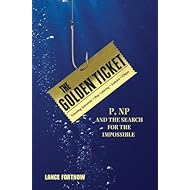The Golden Ticket: P, NP, and the Search for the Impossible
\$16.00
Every computer scientist should have this one. Both a great introduction to the P versus NP problem and a very useful list of NP-complete pr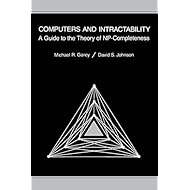Computers and Intractability: A Guide to the Theory of NP-Completeness (Series of Books in the Mathematical Sciences)
Great introduction to theory especially the sections on automata.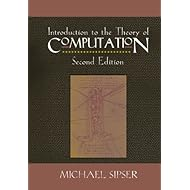Introduction to the Theory of Computation
\$177.00
Great textbook for advanced complexity topics.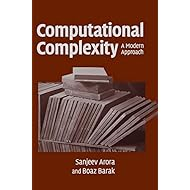Computational Complexity: A Modern Approach
\$60.00
Nice coverage of major results in algorithms.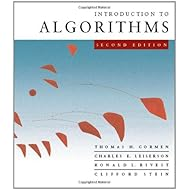Introduction to Algorithms, Second Edition
\$56.00
Excellent surveys on complexity.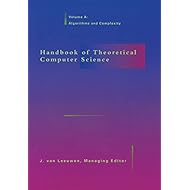Handbook of Theoretical Computer Science, Vol. A: Algorithms and Complexity
\$119.00
An amazingly fun book to read on quantum computing and beyond.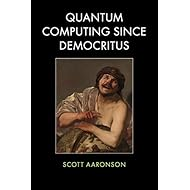Quantum Computing Since Democritus
\$47.00
Readable computational approach to quantum from physicists.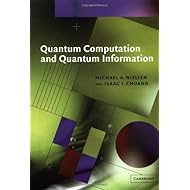Quantum Computation and Quantum Information (Cambridge Series on Information and the Natural Sciences)
\$133.00
Great introduction and reference for Kolmogorov Complexity.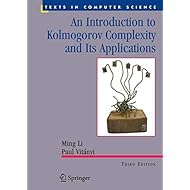An Introduction to Kolmogorov Complexity and Its Applications (Texts in Computer Science)
\$66.00
Computability theory spelled out nicely.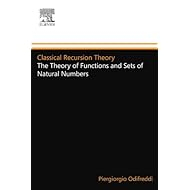Classical Recursion Theory: The Theory of Functions and Sets of Natural Numbers, Vol. 1 (Studies in Logic and the Foundations of Mathematics, Vol. 125) (Volume 125)
\$97.00
Every topic in complexity should have a book like this.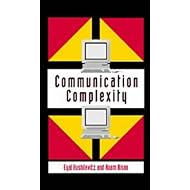Communication Complexity
Computation is manipulating information so useful to have this great book on Information Theory.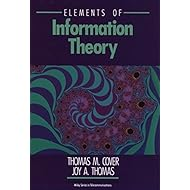Elements of Information Theory (Wiley Series in Telecommunications and Signal Processing)
Probabilistic Method is a powerful tool in complexity. Also has nice section on Chernoff bounds.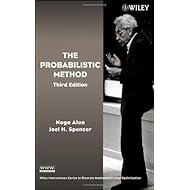The Probabilistic Method
Surprising logical characterizations of computational complexity classes in the well written book by the master himself.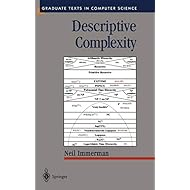Descriptive Complexity (Texts in Computer Science)
\$110.00
Heart this idea list to save it
Inspire others and create your own